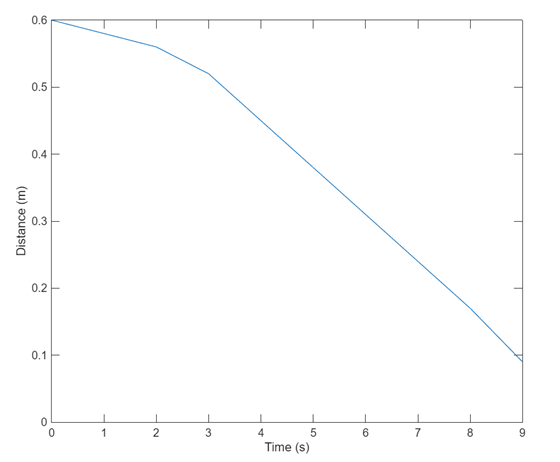# Measure Pulse Width Using NI Devices

This example shows how to measure the width of an active high pulse. A sensor is used to measure distance from a point: the width of the pulse is correlated with the measured distance.

### Create a Counter Input Channel

Create a DataAcquisition, and add a counter input channel with `PulseWidth` measurement type. For this example, use CompactDAQ chassis NI c9178 and module NI 9402 with ID cDAQ1Mod5.

```dq = daq("ni"); ch = addinput(dq, "cDAQ1Mod5", "ctr0", "PulseWidth"); ```

### Determine the Terminal of the Counter Input Channel

To connect the input signal to the correct terminal, examine the `Terminal` property of the channel. The terminal is determined by the hardware.

```ch.Terminal ```
```ans = 'PFI1' ```

### Measure Distance

To determine if the counter is operational, acquire a single scan. The sensor generates a high pulse of width 0.0010 seconds corresponding a distance of one meter.

```1000*read(dq, "OutputFormat", "Matrix") ```
```ans = 5 ```

### Measure Distance over Time

Use the hardware clock to acquire multiple counter measurements over time. NI counter devices require an external clock. By adding an analog input channel for a module on the same chassis, the internal clock is shared with both modules.

```dq = daq("ni"); addinput(dq, "cDAQ1Mod1", "ai0", "Voltage"); addinput(dq, "cDAQ1Mod5", "ctr0", "PulseWidth"); dq.Rate = 1; data = read(dq, seconds(10)); plot(data.Time, 1000*data.cDAQ1Mod5_ctr0); ```## SupportGet trial now